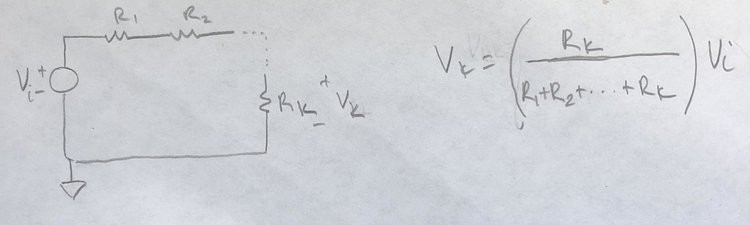# Solving RC Circuit with the Heavyside Operator MethodAssume Vs(t) is equal to 3u(t). Find v(t) as a function of time and find the capacitances of the two capacitors. Assume all initial conditions are 0.

Before we start we should define what u(t) is:It's the step function. Basically when multiplied with another function it just means that the function will start at t = 0, even when it would normally have values below 0. So 3u(t) is:We are also told that the initial conditions are 0, so we don’t have to worry about any energy being stored in the capacitors from before we analyze the circuit, meaning we can just analyze the circuit as given to us in the problem/picture.

To solve this problem, we will use the Heaviside operator method, which gives us a way to solve circuits with capacitors and inductors using their impedances. It states that:For reference, impedance is how much a circuit element opposes the current through it caused by a voltage difference. It is normally denoted as z. In the Heaviside operator method, the impedance of a resistors is just its resistance, R. For capacitors and inductors:What’s given to us in the problem is the impedance. So to find the capacitance, we just use the formula:To find v(t), we first have to solve for what it is in terms of Vs(t). The nice thing about impedances using the Heaviside operator method is that we can treat the impedances just like resistances, meaning that those in series add up, and those in parallel are:Using this we can simplify the circuit to two impedances:NOTE: The voltage across the capacitor and 6 ohm resistor are the same since they are in parallel, so v(t) across the capacitor is the same as across Z2 (since Z2 is just the 12/p and 6 ohm together in parallel).

Once again, we can just treat impedances as resistances, so we can use the voltage divider method on them just like we would with resistors. For reference, the voltage divider method states:NOTE: this only works if the elements are in series, or in other words have the same current running through them. In this case Z1 and Z2 are in series.NOTE: I will be calling v(t) -> v and Vs(t) -> Vs to make the equations less cluttered, but remember that they are equations with respect to time.

Now we can use the formula:## Shayan Raofi

January 11, 2020

View Profile

#### Get in Touch

Thanks! We'll reach out shortly.